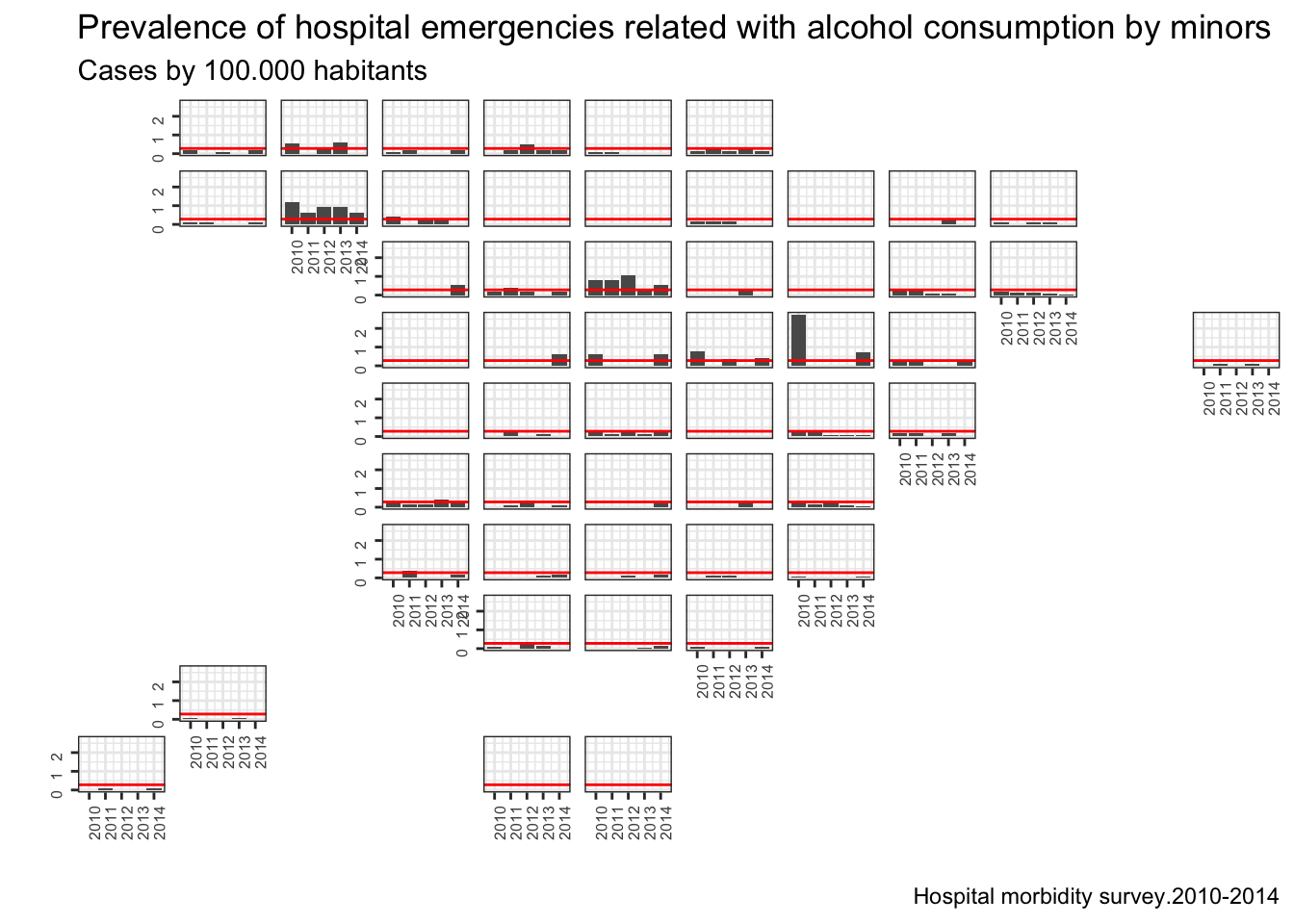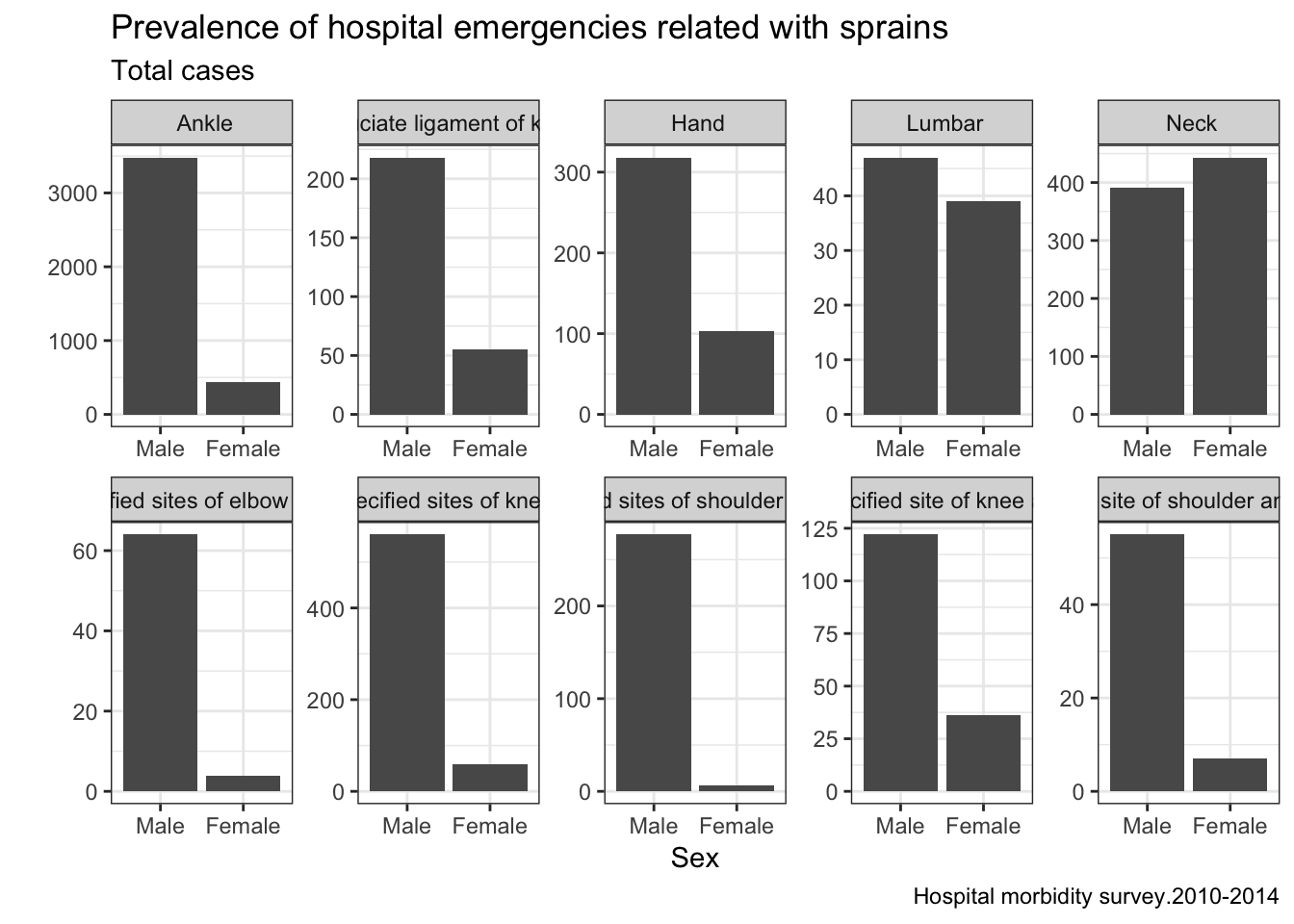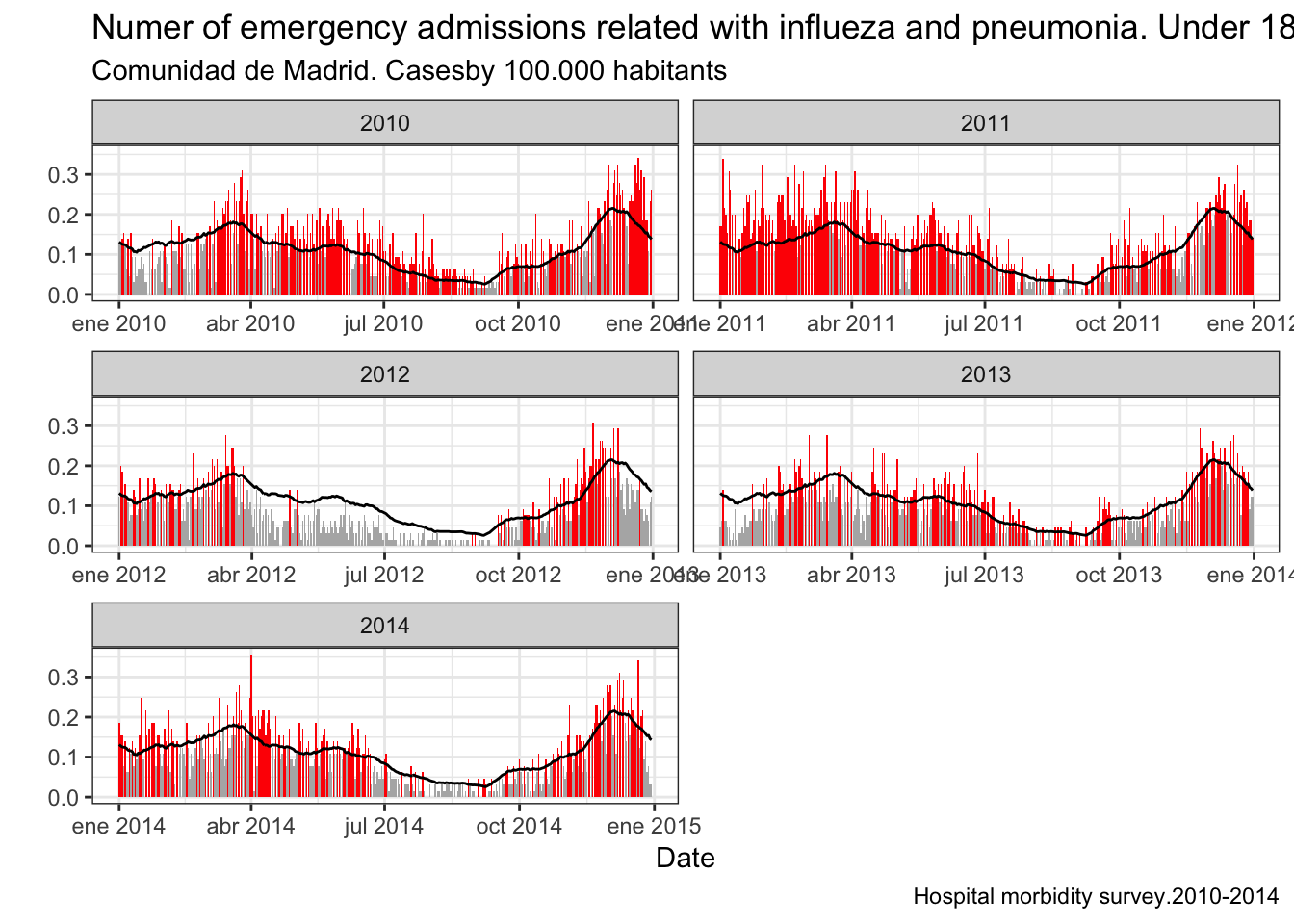# Developing a package to analyze data of the hospital morbidity survey

## Introduction

This post is going to be the most ambitious one so far. Months ago I decided to develope a R package from scratch to learn a little about the process. So I dug up some code I wrote and I got to the work. And of course, for a job like that you want to work properly so I followed R packages by Hadley Wickham

## The data

My package downloads and gives functions to analyze data from the Hospital morbidity survey from Spanish National Institute of Statistics, this data is quite interesting for several reasons:

• Health data is a hot topic these days
• Microdata are available from 1978
• There is enough data to descriptive analysis and forecasting also

One interesting thing in this data is that they have the code corresponding with the International Classification of Diseases which allows a highly detailled analysis. In the middle of the developing process new data were made available. Of course, theses are always good news, but… International Classification of Diseases changed from 9 version to 10. Important disclaimer here, I’m not a doctor and the assumptions I made to translate CIE10 to CIE9 can be wrong.

## The package

The package is hosted in the ROpenSpain gitlab since I thougth it would give visibility and would made it more colaborative. Here you can find a very short intoduction to the package.

## Some descriptive analysis

Once I developed the package (I may write another post with the details) I used it to make some descriptive analysis. This dataset is awesome and a lot of descriptive analysis can be done. Let’s have fun with data!!

### Installing the package

devtools::install_github("rOpenSpain/MorbiditySpainR",quiet = TRUE)
suppressPackageStartupMessages(library(MorbiditySpainR))
suppressPackageStartupMessages(library(lubridate))
suppressPackageStartupMessages(library(dplyr))
suppressPackageStartupMessages(library(ggplot2))
suppressPackageStartupMessages(library(geofacet))

I want to get a dataset light enough to do some descriptive analysis with my personal laptop so I dowload data from 2010 to 2014.

if(!file.exists("data.rds")){
data <- GetMorbiData(y1 = 2010,y2 = 2014)
saveRDS(data,"data.rds")
} else {
}
dplyr::glimpse(data)
## Observations: 23,069,431
## Variables: 10
## $prov_hosp <int> 1, 1, 1, 1, 1, 1, 1, 1, 1, 1, 1, 1, 1, 1, 1, 1, ... ##$ sexo          <int> 1, 1, 1, 1, 2, 2, 1, 1, 1, 1, 2, 1, 1, 2, 2, 2, ...
## $prov_res <int> 1, 1, 1, 1, 1, 1, 1, 1, 1, 1, 1, 1, 1, 1, 1, 1, ... ##$ diag_in       <int> 1, 1, 1, 1, 1, 1, 1, 1, 1, 1, 1, 1, 1, 1, 1, 1, ...
## $diag_ppal <chr> "3019", "3770", "5532", "2851", "8518", "7865", ... ##$ motivo_alta   <int> 1, 1, 1, 1, 1, 1, 1, 1, 1, 1, 4, 1, 3, 1, 1, 3, ...
## $estancia <int> 3, 1, 2, 1, 1, 2, 1, 2, 2, 4, 5, 20, 1, 3, 6, 1,... ##$ cie           <dbl> 9, 9, 9, 9, 9, 9, 9, 9, 9, 9, 9, 9, 9, 9, 9, 9, ...
## $fecha_ingreso <date> 2010-08-29, 2010-04-30, 2010-04-29, 2009-12-31,... ##$ edad          <int> 55, 90, 60, 45, 62, 55, 61, 66, 25, 46, 100, 46,...

### Exploratory analysis

Regrettably we have an issue here in Spain with consumption of alcohol by minors, some times this consumption may end up in a hospital emergency, so let’s find out this in our data.

data <- readRDS("data.rds")
ll <- data %>% filter(year(fecha_ingreso)>=2010)

ll2 <- ll %>% FilterEmergency() %>% filter(edad<18) %>% FilterDiagnosis2(35)
ll2 <- ll2 %>% AddDiagnosis3() %>% ReduceData(provincia = TRUE,date = "year",diag = "diag3")
diag2.35 <- unique(ll2$diag3) diag2.35 <- diag2.35[grepl("alcohol",tolower(diag2.35))] ll2 <- ll2 %>% filter(diag3 %in% diag2.35) ll2 <- ll2 %>% SetPrevalence() ll2 <- ll2 %>% dplyr::group_by(prov,fecha) %>% dplyr::summarise(total=sum(total.prev)) ll2$code <- sprintf("%02d",ll2$prov) ll2$year <- year(ll2$fecha) prov.graf <- geofacet::spain_prov_grid1 ll2 <- full_join(ll2,prov.graf,by="code") ll2.media <- mean(ll2$total,na.rm=TRUE)

g <- ggplot(data=ll2) + geom_bar(aes(x=year,y=total),stat="identity",position="dodge") + geom_hline(yintercept = ll2.media,color="red") + facet_geo(~ name, grid = "spain_prov_grid1") +labs(title="Prevalence of hospital emergencies related with alcohol consumption by minors",subtitle="Cases by 100.000 habitants",caption="Hospital morbidity survey.2010-2014") + xlab("") + ylab("") + theme_bw() + theme(axis.text=element_text(size=6, angle=90),strip.text = element_blank(),strip.background = element_blank())
plot(g)Another descriptive analysis we can do with dataset has the goal of knowing which are the most common sprain in people between 30 and 45 years, splitting resulst by sex.

data <- readRDS("data.rds")
lesiones <- lesiones %>% ReduceData(provincia = TRUE,date = "day",diag = "diag3",sex=TRUE)

lesiones.y <- lesiones %>% group_by(diag=diag3,sex=sex) %>% summarise(total=sum(total))
esguinces <- lesiones.y %>% group_by(diag) %>% summarise(tt=sum(total)) %>% top_n(10,tt)
esguinces <- esguinces$diag lesiones.y <- lesiones.y %>% filter(diag %in% esguinces) lesiones.y$sex <- factor(x = lesiones.y$sex,labels = c("Male","Female")) g2 <- ggplot(data=lesiones.y) + geom_bar(aes(x=sex,y=total),stat="identity",position="dodge") + facet_wrap(~diag,nrow = 2,ncol = 5,scales = "free") + labs(title="Prevalence of hospital emergencies related with sprains",subtitle="Total cases",caption="Hospital morbidity survey.2010-2014") + xlab("Sex") + ylab("") + theme_bw() plot(g2)The next analysis imitates the famous Myth busters with data and answer the question of whether they are born more babies with full moon. data <- readRDS("data.rds") partos <- data %>% FilterEmergency() %>% FilterDiagnosis2(77) %>% ReduceData(provincia = FALSE,date = "day",sex = FALSE) library(lunar) partos$phase <- lunar.phase(partos$fecha,name=8) partos <- partos %>% group_by(phase) %>% summarise(total=sum(total)) g3 <- ggplot(partos) + geom_bar(aes(x=phase,y=total),stat="identity",position = "dodge") + labs(title="Number of births and moon phase",subtitle="Total cases",caption="Hospital morbidity survey.2010-2014") + xlab("Fase Lunar") + ylab("") + theme_bw() plot(g3)The last example builds a temporal series of hospital admissions of minors related with repiratorial diseases (flu and pneumonia) in Madrid. With this temporal serie we can construct a mean serie and compare them both to find epimediological episodes. library(zoo) data <- readRDS("data.rds") ll.gripe <- data %>% FilterProvincia(28) %>% FilterEmergency() %>% dplyr::filter(edad<18) %>% FilterDiagnosis2(57) %>% ReduceData(provincia = TRUE,date="day",sex = FALSE) %>% SetPrevalence(pop = "total") ll.gripe$yday <- yday(ll.gripe$fecha) ll.gripe.clim <- ll.gripe %>% dplyr::group_by(yday) %>% dplyr::summarise(mean=mean(total.prev,na.rm=TRUE)) ll.gripe.rollmean <- bind_rows(ll.gripe.clim,ll.gripe.clim,ll.gripe.clim) ll.gripe.rollmean <- rollmean(ll.gripe.rollmean$mean,15,fill=NA)[367:732]
ll.gripe.clim$mean <- ll.gripe.rollmean # g4 <- ggplot(ll.gripe.clim) + geom_line(aes(x=yday,y=mean)) ll.gripe <- full_join(ll.gripe,ll.gripe.clim,by="yday") ll.gripe$color <- ifelse(test = ll.gripe$total.prev>ll.gripe$mean,"si","no")
cols <- c("no" = "gray70", "si" = "red")
ll.gripe <- ll.gripe %>% dplyr::filter(year(fecha)>=2010)
g4 <- ggplot(ll.gripe) + geom_bar(aes(x=fecha,y=total.prev,fill=color),stat="identity",position = "dodge") + geom_line(aes(x=fecha,y=mean)) + facet_wrap(~year(fecha),ncol=2,scales = "free_x") + scale_fill_manual(values=cols,guide=FALSE) + labs(title="Numer of emergency admissions related with influeza and pneumonia. Under 18 years",subtitle="Comunidad de Madrid. Casesby 100.000 habitants",caption="Hospital morbidity survey.2010-2014") + xlab("Date") + ylab("") + theme_bw()
plot(g4)## Coming next

• Predictive anlysis
• Big data